Published: 03 November 2014

# A time-based model for random vibration fatigue analysis

Shan Jiang1
Wei Zhang2
1, 2Science and Technology on Reliability and Environmental Engineering Laboratory, School of Reliability and Systems Engineering, Beihang University, Beijing, China
Corresponding Author:
Wei Zhang
Views 88

## 1. Introduction

Vibration fatigue theory has been introduced to deal with the fatigue behavior under any kinds of arbitrary vibration loading. Many structures components are frequently subjected to vibration loading of random nature. Since fatigue is one of the predominant mechanical failure causes, fatigue life prediction has become a necessary subject in many researches [1-3]. The approach of predicting fatigue life could be divided into frequency domain and time domain. The time domain methods are based on the cycle counting and cumulative damage rules . The identification of cycles is usually carried out through the rainflow counting method . And then the Miner damage accumulation rule is used to perform the accumulation of damage. The time domain has been widely accepted to predict the fatigue life. However, when a component is subject to variable amplitude loading, the cycle calculation will be very time consuming. In addition, the variable load interaction has the influence on the fatigue crack growth behavior which the most of the existing time domain methods cannot account for . Therefore, our investigation in this paper focuses on the time-based model development aim to predict the fatigue crack behavior of components subjected to arbitrary variable amplitude loading.

Many models have been proposed to predict the fatigue crack propagation under variable amplitude loading. Elber proposed the approach to describe the crack growth behavior, which considers crack closure . Wheeler  and Willenborg  presented a series of models based on the plastic zone size ahead of the crack tip. The fatigue crack growth computer program developed by Forman et al. could be used to estimate for constant and variable amplitude loading . Most studies involve the nonlinear analysis of cyclic plasticity and crack contact analysis, which causes the computing process of the random variable amplitude loadings situation extremely complicated.

In many recent studies, the CTOD variation concept has been indicated to correlate with the fatigue crack growth rate under cyclic loadings [11, 12]. Liu, Lu and Xu proposed a theoretical CTOD formulation under variable amplitude loading . In addition, Zhang and Liu performed a state-of-art in-situ SEM testing and presented a virtual crack annealing model to calculate the crack closure which has the effect on the CTOD variation [12, 13]. Based on that, Zhang and Liu developed a time-based fatigue crack growth model to predict the fatigue crack behavior under variable amplitude loading, which combine the CTOD variation estimation with the crack closure model . Through this model, the CTOD variation at any loading level can be obtained.

The paper is organized as follows. First, the model to calculate the CTOD variation which is combined with the crack closure model and the fatigue damage evolution function is briefly derived. Based on this method, the fatigue crack growth rate can be estimated. Next, the fatigue testing of Al 7075-T6 under “Christmas Tree” loading has been performed to verify this formulation. By using the “Christmas Tree” loading, the high ratio and low ratio situation will be discussed. The predictions of the proposed approach match the experimental data very well. Moreover, the method will be simulated under random loading. Finally, some conclusions are given based on the current investigation.

## 2. Methodology

It is proven that CTOD variation correlates with the fatigue crack propagation. The analytical solution of CTOD could be expressed as :

1
$\delta =\frac{{K}^{2}}{E{\sigma }_{y}}=\frac{{\sigma }^{2}\pi a{Y}^{2}}{E{\sigma }_{y}},$

where $E$ is the Young’s modulus, and ${\sigma }_{y}$ is the yield strength. $K$ is the stress intensity factor (SIF), which can be expressed as :

2
$\begin{array}{l}K=\sigma \sqrt{\pi a}\cdot Y,\\ Y\left(a/w\right)=1.12-0.23\left(a/w\right)+10.55\left(a/w{\right)}^{2}-21.22\left(a/w{\right)}^{3}+30.39\left(a/w{\right)}^{4},\end{array}$

where $a$ is the crack length and $w$ is the plate width.

A general mathematic calculation of CTOD under random cyclic loading is derived in paper . And then the crack closure is considered. It is proven that the crack closure is an important factor of the fatigue crack growth behavior through the fatigue testing and theoretical analysis [12, 13]. Therefore, Zhang and Liu modified the formulation above . Based on a stationary crack, the mathematic estimation of CTOD variation with crack closure during the reloading path and unloading path can be expressed as :

3
${\delta }_{loading}$
$=\left\{\begin{array}{ll}\frac{{K}^{2}}{E{\mathrm{\sigma }}_{y}},& K>{K}_{\mathrm{m}\mathrm{a}\mathrm{x},mem},\\ {\delta }_{min,m-1}+\frac{\left(K-max\left({K}_{min,m-1},{K}_{ref,m-1}\right){\right)}^{2}}{2E{\sigma }_{y}}H\left(K-{K}_{ref,m-1}\right),& \begin{array}{c}{K}_{max,mem}\ge K,\\ K\ge {K}_{max,m-1},\end{array}\\ {\delta }_{min,m}+\frac{\left(K-max\left({K}_{min,m},{K}_{ref,m}\right){\right)}^{2}}{2E{\sigma }_{y}}H\left(K-{K}_{ref,m}\right),& {K}_{\mathrm{m}\mathrm{a}\mathrm{x},m-1}\ge K,\end{array}\right\$
${\delta }_{unloading}$
$=\left\{\begin{array}{l}{\delta }_{max,m-1}-\frac{\left({K}_{max,m-1}-max\left(K,{K}_{ref,m-1}\right){\right)}^{2}}{2E{\sigma }_{y}}H\left({K}_{max,m-1}-{K}_{ref,m-1}\right),K\le {K}_{min,m-1},\\ {\delta }_{max,m}-\frac{\left({K}_{max,m}-\mathrm{m}\mathrm{a}\mathrm{x}\left(K,{K}_{ref,m-1}\right){\right)}^{2}}{2E{\sigma }_{y}}H\left({K}_{max,m}-{K}_{ref,m}\right),K\ge {K}_{min,m-1},\end{array}\right\$

where ${K}_{ref,m}$ indicates the crack closure loading level at the $m$th peak or valley. $H$ is the Heaviside step function. If the $K$ or ${K}_{max}$ is smaller than ${K}_{ref}$, the crack will close and CTOD will remain constant.

All the derivation above is based on the stationary crack. For the practical crack, the total CTOD variation considering the crack growth can be written as :

4
$d\mathrm{\delta }=\frac{\partial \mathrm{\delta }}{\partial \mathrm{\sigma }}d\mathrm{\sigma }+\frac{\partial \mathrm{\delta }}{\partial a}da.$

Therefore, the general calculation for CTOD can be expressed as :

5
$d{\delta }_{loading}$
$=\left\{\begin{array}{l}\frac{2\sigma \pi a{Y}^{2}}{E{\sigma }_{y}}d\sigma +\frac{{\sigma }^{2}\pi {Y}^{2}}{E{\sigma }_{y}}da,\sigma >{\sigma }_{max,mem},\\ \begin{array}{c}\frac{\left(\sigma -\mathit{max}\left({\sigma }_{min,m-1},{\sigma }_{ref,m-1}\right)\right)\pi a{Y}^{2}}{E{\sigma }_{y}}d\sigma +\frac{\left(\sigma -max\left({\sigma }_{min,m-1},{\sigma }_{ref,m-1}\right){\right)}^{2}\pi {Y}^{2}}{2E{\sigma }_{y}}da,\\ {\sigma }_{max,m-1}\ge \sigma ,\end{array}\\ 0,H\left(\sigma -{\sigma }_{ref}\right)=0,\end{array}\right\$
$d{\delta }_{unloading}=\left\{\begin{array}{l}-\frac{\left({\sigma }_{max,m-1}-\mathit{max}\left(\sigma ,{\sigma }_{ref,m-1}\right)\right)\pi a{Y}^{2}}{E{\sigma }_{y}}d\sigma ,\sigma \le {\sigma }_{min,m-1},\\ -\frac{\left({\sigma }_{max,m}-\mathit{max}\left(\sigma ,{\sigma }_{ref,m-1}\right)\right)\pi a{Y}^{2}}{E{\sigma }_{y}}d\sigma ,\sigma \ge {\sigma }_{min,m-1},\\ 0\begin{array}{llll},& & & H\left({\sigma }_{max}-{\sigma }_{ref}\right)=0.\end{array}\end{array}\right\$

In order to predict the fatigue crack propagation, the simple mathematical model is present in paper . The instantaneous crack growth rate can be expressed as :

6
$\frac{da}{dt}=f\left({K}_{\mathrm{m}\mathrm{a}\mathrm{x}},\mathrm{\Delta }K,\delta \right)=\left\{\begin{array}{l}\frac{A\cdot \mathrm{\Delta }K\cdot {K}_{max}^{}}{2\sqrt{{\delta }_{}-{\delta }_{ref}}}\frac{d\delta }{dt},{\delta }_{}-{\delta }_{ref}>0,\\ 0\begin{array}{lll},& & \text{otherwise}\end{array},\end{array}\right\$

where $A$ is a fitting parameter. Based on the formulation above, the crack length incremental at any point during the cyclic loading can be written as :

7
$\mathrm{\Delta }a={\int }_{{\sigma }_{t0}}^{{\sigma }_{t1}}‍f\left({K}_{max},\Delta K,\delta \right)dt.$

## 3. Model validation

In this section, the model above will be validated through comparing the predictions with the experimental data. The Fig. 1 shows the type of vibration loading spectrum, which could be considered as the superposition of two loading spectra.

Therefore, the “Christmas Tree” loading spectrum is employed to verify the model. Zhang and Liu provided fatigue test data for edge-cracked 7075-T6 aluminum alloy specimen under variable amplitude loading . The geometric dimensions of the specimen are respectively: thickness$=$ 4.7 mm, width$=$40 mm. The geometric factor of the stress intensity factor for this specimen is:

8
$Y=\frac{1}{\sqrt{\pi \alpha }}\left(\frac{2+\alpha }{\left(1-\alpha {\right)}^{3/2}}\right)\left(0.886+4.64\alpha -13.32{\alpha }^{2}+14.72{\alpha }^{3}-5.6{\alpha }^{4}\right).$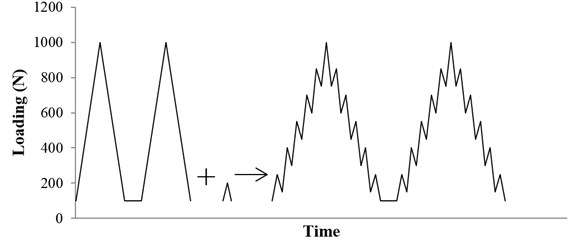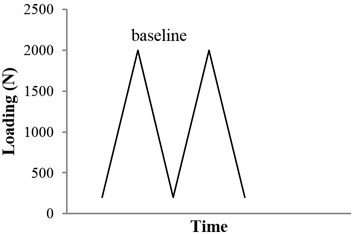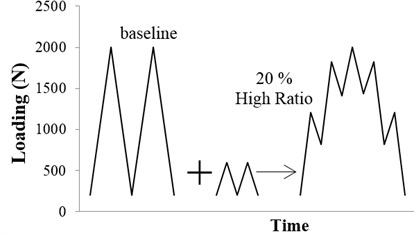b) 20 % high ratio spectrum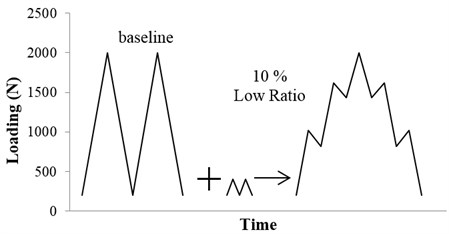c) 10 % low ratio spectrum

Fig. 3Comparison of the model predictions with experimental results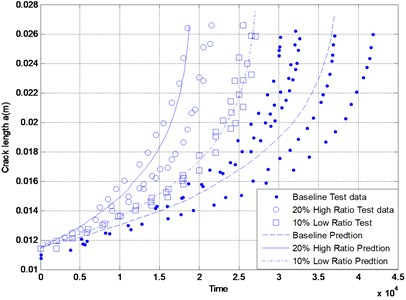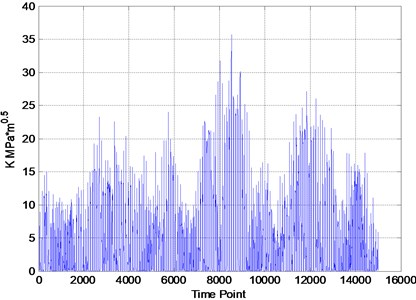The experimental loading spectra are shown in Fig. 2. The typical arbitrary load spectrum situation is included in the “Christmas Tree” spectra. The vibration is treated as loading spectrum to affect the fatigue crack propagation behavior.

The model predictions are compared to the test data in Fig. 3. It is obvious that the predictions match the experimental data very well. The present formulation provides a good estimate of fatigue crack growth under arbitrary amplitude loading.

In this section, the simulation of the model above will be illustrated. The variable amplitude loading spectrum is shown in Fig. 4.

According to the Eq. (5), the CTOD variation under variable amplitude loading is calculated and shown in the Fig. 5. When the $K$ is less than ${K}_{ref}$, the crack is closed and the CTOD remains constant.

More detailed information can be obtained in the $N$-$a$ curve in Fig. 6, which shows the crack growth rate under the variable amplitude loading.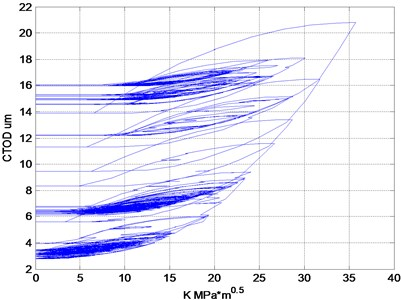Fig. 6Simulation of the model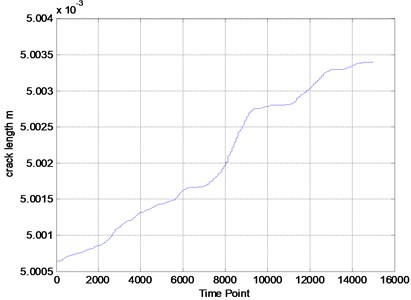## 4. Conclusions

The fatigue crack growth behavior under random vibration loading is usually accounted for by analyzing the fatigue properties. The vibration is treated as the loading history or loading spectrum to affect the crack propagation. Therefore, the time-based fatigue crack growth model is described in this paper to predict the fatigue crack growth under variable amplitude loading. This model is based on the CTOD variation, which is combined with the crack closure. The following conclusions can be drawn:

The time-based method is proven to predict the fatigue life under variable amplitude loading easily, accurately and successfully through the fatigue testing and the simulation validation. Furthermore, this model avoids complex calculation process and cycle counting, which is different from the existing method.

The model validation using “Christmas Tree” is performed and very good correlations are observed between model predictions and experimental observations.

During the vibration cycles, the loading frequency has effects on the crack growth. However, the frequency factor is not considered in this investigation. In the future, the method should be extended by taking account of the frequency influence.